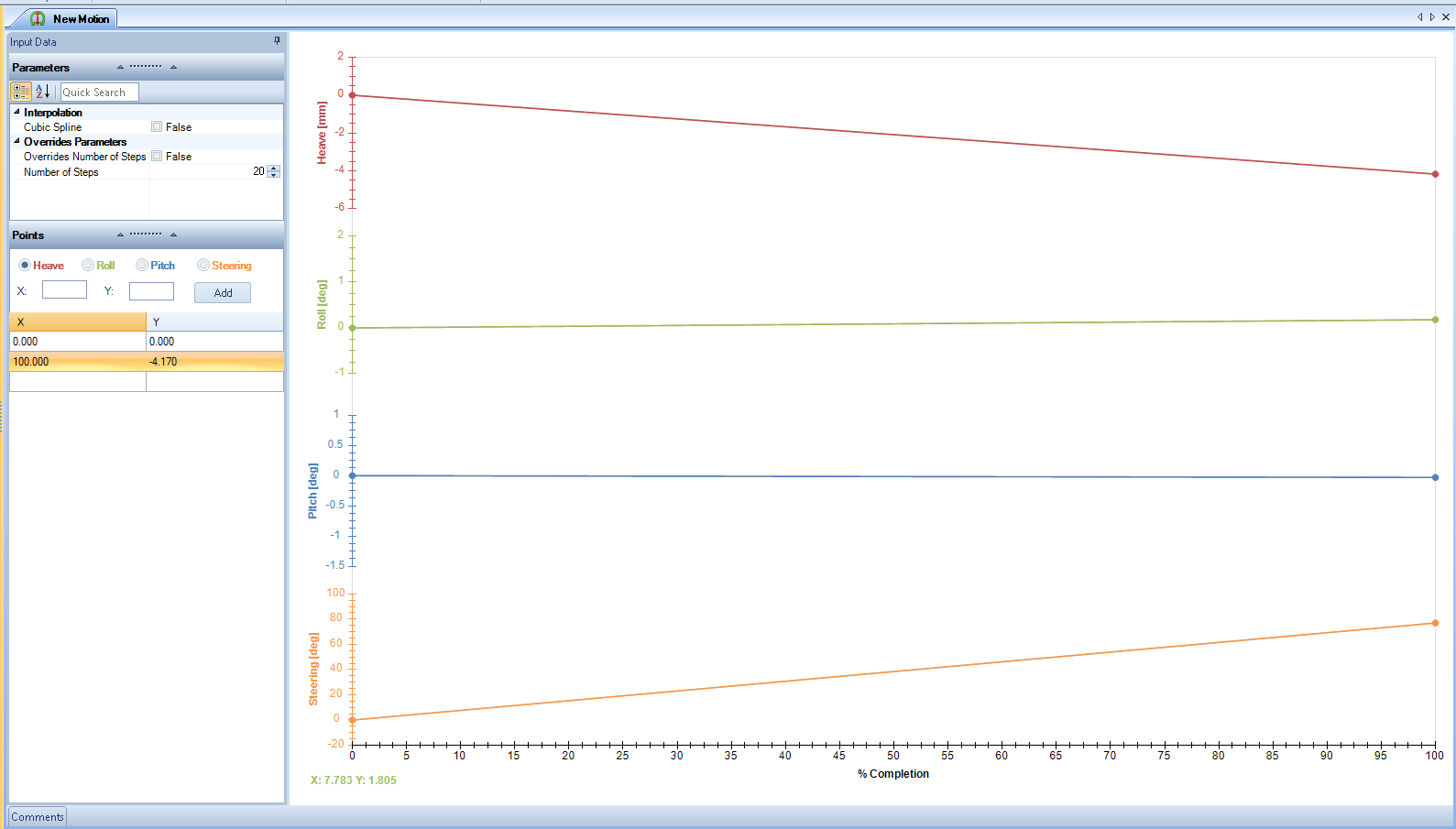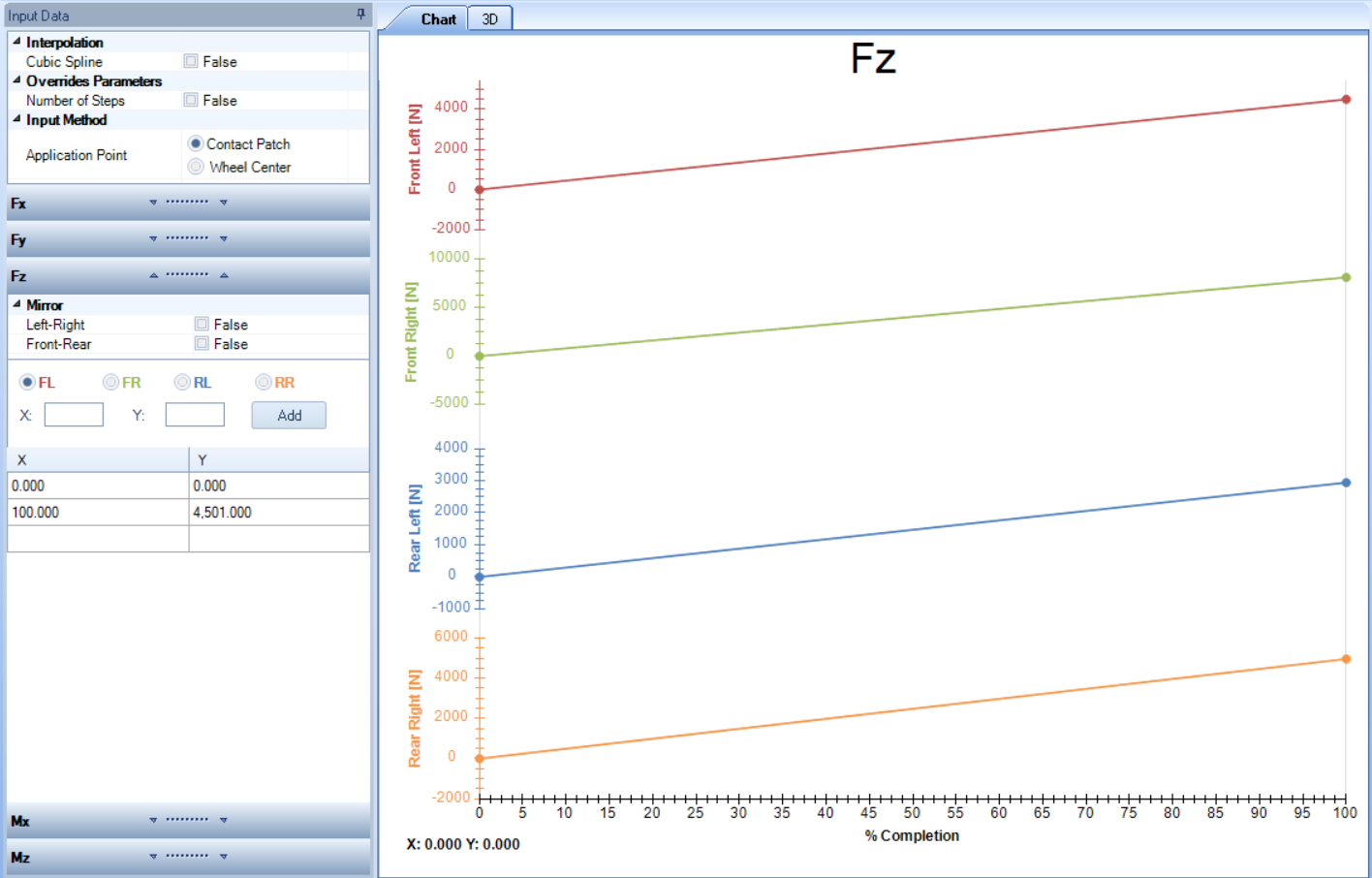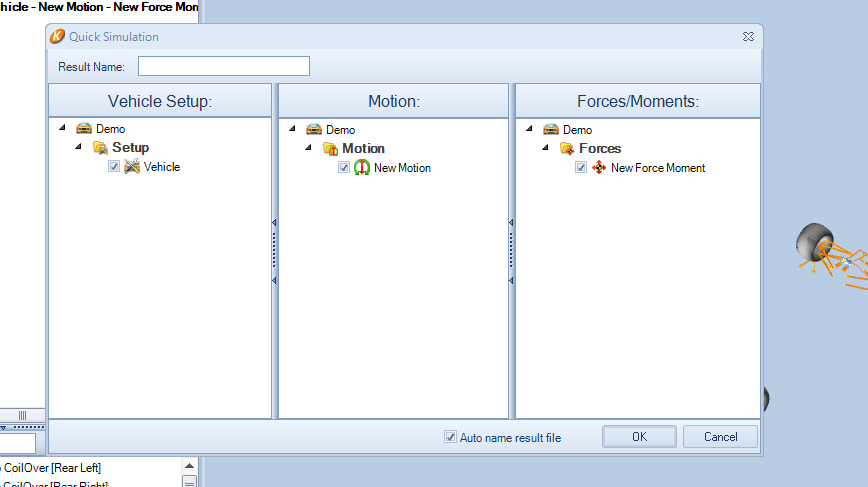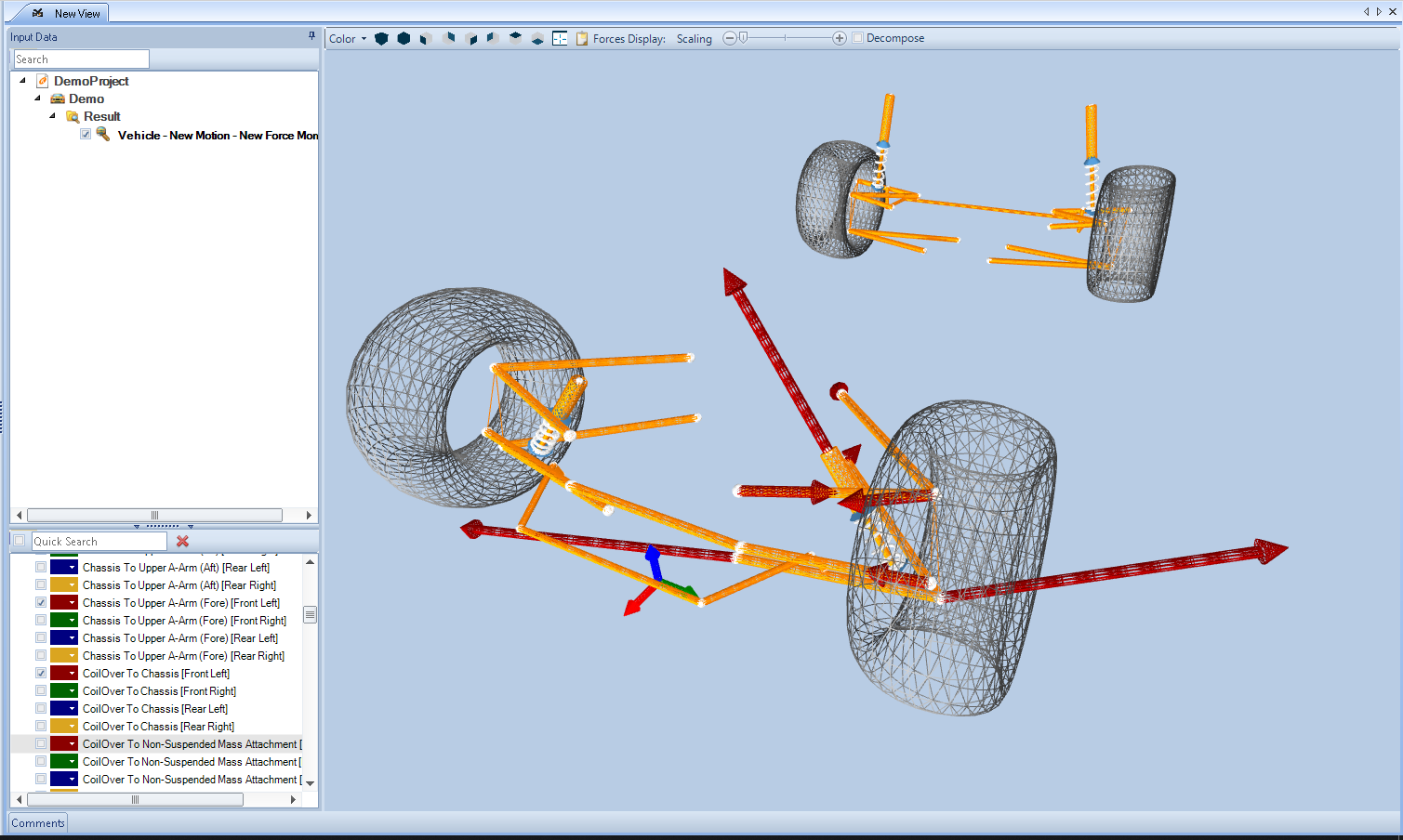### OptimumKinematics Forces Module – A Case Study

Posted at June 25, 2019 | By : | Categories : OptimumKinematics Forces Module,Uncategorized | 0 Comment

OptimumKinematics aids users in the design and setup of their suspension in an intuitive and straightforward manner, providing useful analysis tools with a user-friendly visual interface. OptimumKinematics is the easiest way for engineers to understand and maximize the performance of their suspension kinematics.

We know that being able to understand not only your suspension kinematic behavior, but also evaluating forces at each pickup point due to external forces becomes essential when designing or analyzing a suspension system. The currently available tools in the market are usually time consuming both in terms of acquiring inputs, as well as modeling your system.

### Forces and Moments

With our Forces Module, which can be incorporated into OptimumKinematics, the task of analyzing forces in the suspension pickup points becomes straightforward, simple, and conclusive. Creating a suspension item and running a simulation with motion and forces takes just minutes. The user has the task of choosing a suspension type (and we have dozens of modeled suspension types), entering the suspension pickup points and defining a motion and a force input – all the rest is done by the software.

To showcase this feature, a braking and cornering combined maneuver is simulated. The best option would be the case where the user knows all the force inputs at the contact patch, but this is usually not the case. By knowing some properties of the vehicle the user can estimate what should be the loads at each corner for a given lateral and longitudinal accelerations. In order to easily estimate the loads for given accelerations, we created an Excel spreadsheet tool.

In figure 1, on the top left corner of the spreadsheet we have the inputs of the car. On the bottom left corner, we can define what is the maneuver the car is taking by entering what is the longitudinal and lateral accelerations, and the speed. In this example, a lateral acceleration of 2g and a braking longitudinal acceleration of 1g are applied, representing a maneuver of combined cornering and braking. On the right side of the spreadsheet we have the simulation results for motion (heave, roll, pitch, and steering), and forces and moments at the contact patch (vertical, longitudinal, lateral, and self-aligning torque).

### Defining the Motion, Forces and Moments

Once we defined the motion and forces that we will use as inputs for the simulation, the user can create the motion and the forces items in Optimum Kinematics. When creating the motion input, we define the initial position of the suspension in the simulation as being its static position, and to do this we should keep the value 0 for heave, roll, and pitch in the first step of the simulation. We will set the final state of the motion in the simulation as the motion we calculated with the spreadsheet (heave of 8.54 mm, roll of 0.42 deg, pitch of -0.35 deg, and steering of 70 deg). By doing so, we are simulating the vehicle starting from 0 lateral and longitudinal acceleration (static position) until it reaches our target values, in this case, 1.4 g of lateral acceleration, and 1.4 g of longitudinal acceleration in braking.Figure 2 – Window where the user can define the heave, roll, pitch and steering motion

Once the motion is defined the next step is to define the forces. The user has the ability of defining if the forces are applied at the wheel center or at the contact patch, and also apply different forces and moments at each corner of the suspension. If there is a symmetry in the force inputs between left and right, or front and rear, OptimumKinematics will copy the forces from one side/axle to the other as requested by the user. Figure 3 and 4 show, as examples, the vertical force and self-aligning torque input for each corner as defined in the force item. Following the same reasoning as for the motion item, the forces and moments start from zero, up to the values found with our tool.Figure 3 – Window where the user can define the forces and moments: Fx, Fy, Fz, Mx, Mz. In this image it is highlighted the vertical forceFigure 4 – Same window as figure 3, highlighting the self-aligning torque (Mz)

### Simulation Time

Once the suspension, motion, and forces are defined, it’s time to run the simulation, as shown in figure 5. With OptimumKinematics, it is possible to perform a simulation with only the motion input, or the force input, or both. The user is also able to run any combination of vehicles, motion inputs, and force inputs, meaning that you can easily simulate various motions and load cases at once.### Visualizing The Results

After the simulation is completed, the user can visualize the forces and moments of each suspension pickup point, as shown in figure 6, making it easy to have a visual check of how the forces and moments are at each point of interest. The user has to ability to choose which points to display its forces and moments.Figure 6 – Visual Representation of the Forces for the Front Left Suspension

Alternatively, the user can create a table and select the pickup points of interest. For every connected pair of bodies in a suspension, 2 sets of force channels and 2 sets of moment channels are created, one set with forces (and moments) from “body A” to “body B” and the other one from “body B” to “body A”. These forces and moments are provided both as a total value, and as split into its X, Y, and Z coordinates. In figure 7 we have selected pickup points of the front left corner (lower a-arm (fore) to chassis; lower a-arm (aft) to chassis; upper a-arm (fore) to chassis; and upper a-arm (aft) to chassis). If we look at the last step, step , that corresponds to our maximum load case, we can see that the highest load is happening at the lower a-arm (fore) to chassis.

### Key Points

In this example it was demonstrated that OptimumKinematics can calculate in a short amount of time the loads on each suspension pickup point. This can be further expanded, and the user can create a set of pre-established load cases (maneuvers): maximum pure lateral, maximum pure longitudinal, or a combination of each, and the acceleration for each of them can come from real data of your car, if you wish, and our spreadsheet tool can help you to calculate the required loads at each contact patch to be used as inputs. A set of different suspension setups can also be created and for each setup the user can simulate its defined load cases. The results giving you the forces and moments at each pickup point can be used as inputs in FEA software simulations, making it straightforward to acquire the inputs for your structural simulations, and helping the engineers in designing their vehicle.